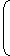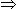# Percentage

Percent means ‘per every hundred’. 5% means ‘5 per 100’.
In other words, percentage is a fraction with denominator as 100.

Percentage is denoted as P.C. or %. Ex: 70 percent = 70%.

Convert Percentage into Fraction:
Step 1: The number is divided by 100.

Convert Fraction Into Percentage:
Step 1: Multiply fraction by 100.
Step 2: Put a ‘%’ sign.

Convert Percentage into Decimal:

Convert Decimal Into Percentage:
0.25 = (0.25 × 100)%  = 25%
1.50 = (1.50 × 100)% = 150%

Some Useful Formulas:

#### Number of valid votes = 80% of 7500 = 6000.Valid votes polled by other candidate = 45% of 6000
 =45 x 6000= 2700. 100

2.Gauri went to the stationers and bought things worth Rs. 25, out of which 30 paise went on sales tax on taxable purchases. If the tax rate was 6%, then what was the cost of the tax free items?

Let the amount taxable purchases be Rs. x.
 Then, 6% of x = 30 100x =30 x 100= 5. 100 6Cost of tax free items = Rs. [25 - (5 + 0.30)] = Rs. 19.70

3.A batsman scored 110 runs which included 3 boundaries and 8 sixes. What percent of his total score did he make by running between the wickets?Required percentage =50 x 100% = 45 5 % 110 11